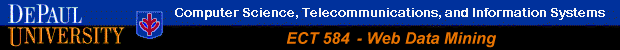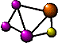K-Means Clustering in WEKA

 The following guide is based WEKA version 3.4.1 Additional resources on WEKA, including sample data sets can be found from the official WEKA Web site.

This example illustrates the use of k-means clustering with WEKA The sample data set used for this example is based on the "bank data" available in comma-separated format (bank-data.csv). This document assumes that appropriate data preprocessing has been perfromed. In this case a version of the initial data set has been created in which the ID field has been removed and the "children" attribute has been converted to categorical (This, however, is not necessary for clustering).

The resulting data file is "bank.arff" and includes 600 instances. As an illustration of performing clustering in WEKA, we will use its implementation of the K-means algorithm to cluster the cutomers in this bank data set, and to characterize the resulting customer segments.

Figure 34 shows the main WEKA Explorer interface with the data file loaded.

Figure 34

Some implementations of K-means only allow numerical values for attributes. In that case, it may be necessary to convert the data set into the standard spreadsheet format and convert categorical attributes to binary. It may also be necessary to normalize the values of attributes that are measured on substantially different scales (e.g., "age" and "income"). While WEKA provides filters to accomplish all of these preprocessing tasks, they are not necessary for clustering in WEKA . This is because WEKA SimpleKMeans algorithm automatically handles a mixture of categorical and numerical attributes. Furthermore, the algorithm automatically normalizes numerical attributes when doing distance computations. The WEKA SimpleKMeans algorithm uses Euclidean distance measure to compute distances between instances and clusters.

To perform clustering, select the "Cluster" tab in the Explorer and click on the "Choose" button. This results in a drop down list of available clustering algorithms. In this case we select "SimpleKMeans". Next, click on the text box to the right of the "Choose" button to get the pop-up window shown in Figure 35, for editing the clustering parameter.

Figure 35

In the pop-up window we enter 6 as the number of clusters (instead of the default values of 2) and we leave the value of "seed" as is. The seed value is used in generating a random number which is, in turn, used for making the initial assignment of instances to clusters. Note that, in general, K-means is quite sensitive to how clusters are initially assigned. Thus, it is often necessary to try different values and evaluate the results.

Once the options have been specified, we can run the clustering algorithm. Here we make sure that in the "Cluster Mode" panel, the "Use training set" option is selected, and we click "Start". We can right click the result set in the "Result list" panel and view the results of clustering in a separate window. This process and the resulting window are shown in Figures 36 and 37.

Figure 36       Figure 37

The result window shows the centroid of each cluster as well as statistics on the number and percentage of instances assigned to different clusters. Cluster centroids are the mean vectors for each cluster (so, each dimension value in the centroid represents the mean value for that dimension in the cluster). Thus, centroids can be used to characterize the clusters. For example, the centroid for cluster 1 shows that this is a segment of cases representing middle aged to young (approx. 38) females living in inner city with an average income of approx. \$28,500, who are married with one child, etc. Furthermore, this group have on average said YES to the PEP product.

Another way of understanding the characteristics of each cluster in through visualization. We can do this by right-clicking the result set on the left "Result list" panel and selecting "Visualize cluster assignments". This pops up the visualization window as shown in Figure 38.

Figure 38

You can choose the cluster number and any of the other attributes for each of the three different dimensions available (x-axis, y-axis, and color). Different combinations of choices will result in a visual rendering of different relationships within each cluster. In the above example, we have chosen the cluster number as the x-axis, the instance number (assigned by WEKA) as the y-axis, and the "sex" attribute as the color dimension. This will result in a visualization of the distribution of males and females in each cluster. For instance, you can note that clusters 2 and 3 are dominated by males, while clusters 4 and 5 are dominated by females. In this case, by changing the color dimension to other attributes, we can see their distribution within each of the clusters.

Finally, we may be interested in saving the resulting data set which included each instance along with its assigned cluster. To do so, we click the "Save" button in the visualization window and save the result as the file "bank-kmeans.arff". The top portion of this file is depicted in Figure 39.

Figure 39

Note that in addition to the "instance_number" attribute, WEKA has also added "Cluster" attribute to the original data set. In the data portion, each instance now has its assigned cluster as the last attribute value. By doing some simple manipulation to this data set, we can easily convert it to a more usable form for additional analysis or processing. For example, here we have converted this data set in a comma-separated format and sorted the result by clusters. Furthermore, we have added the ID field from the original data set (before sorting). The results of these steps can be seen in the file "bank- kmeans.csv".Return to Main Page